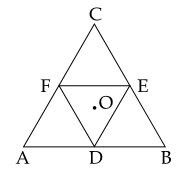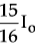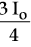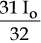#### Moment of inertia of an equilateral triangular lamina ABC, about the axis passing through its centre O and perpendicular to its plane is Io as shown in the figure.  A cavity DEF is cut out from the lamina, where D, E, F are the mid points of the sides.  Moment of inertia of the remaining part of lamina about the same axis is :Option 1)Option 2)Option 3)Option 4)As we learnt in

Perpendicular Axis theorem -(for a body in XY plane )

- wherein= moment of inertia about z axis.:moment of inertia about x & y  axis in the plane of body respectively.

According to the theorem of perpendicular axes.  Moment of inertia of triangle (ABC)Moment of inertia of cavity (DEF)Therefore, Remaining partOption 1)This is an incorrect option.

Option 2)This is the correct option.

Option 3)This is an incorrect option.

Option 4)This is an incorrect option.

#### perimeter